Next: Acoustics Up: Types of analysis Previous: Direct integration dynamic analysis   Contents

### Heat transfer

In a heat transfer analysis, triggered by the *HEAT TRANSFER procedure card, the temperature is the independent degree of freedom. In essence, the energy equation is solved subject to temperature and flux boundary conditions (). For steady-state calculations it leads to a Laplace-type equation.

The governing equation for heat transfer reads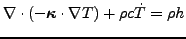(307)

where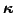contains the conduction coefficients,is the density,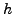the heat generation per unit of mass and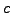is the specific heat.

The temperature can be defined using the *BOUNDARY card using degree of freedom 11. Flux type boundary conditions can consist of any combination of the following:

1. Concentrated flux, applied to nodes, using the *CFLUX card (degree of freedom 11)
2. Distributed flux, applied to surfaces or volumes, using the *DFLUX card
3. Convective flux defined by a *FILM card. It satisfies the equation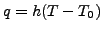(308)

where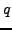is the a flux normal to the surface,is the film coefficient,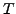is the body temperature and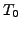is the environment temperature (also called sink temperature). CalculiX can also be used for forced convection calculations, in which the sink temperature is an unknown too. This applied to all kinds of surfaces cooled by fluids or gases.

4. Radiative flux defined by a *RADIATE card. The equation reads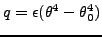(309)

whereis a flux normal to the surface,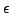is the emissivity,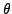is the absolute body temperature (Kelvin) and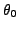is the absolute environment temperature (also called sink temperature). The emissivity takes values between 0 and 1. A zero value applied to a body with no absorption nor emission and 100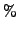reflection. A value of 1 applies to a black body. The radiation is assumed to be diffuse (independent of the direction of emission) and gray (independent of the emitted wave length). CalculiX can also be used for cavity radiation, simulating the radiation interaction of several surfaces. In that case, the viewfactors are calculated, see also  for the fundamentals of heat transfer and  for the calculation of viewfactors.

The calculation of viewfactors involves the solution of a four-fold integral. By using analytical formulas derived by Lambert this integral can be reduced to a two-fold integral. This is applied in CalculiX right now: the interacting surfaces are triangulated and the viewfactor between two triangles is calculated by taking a one-point integration for the base triangle (in the center of gravity) and the analytical formula for the integration over the other triangles covering a hemisphere about the base triangle. One can switch to a more accurate integration over the base triangle by increasing the variable factor'' in subroutine radmatrix, look at the comments in that subroutine. This, however, will increase the computational time.

For a heat transfer analysis the conductivity coefficients of the material are needed (using the *CONDUCTIVITY card) and for transient calculations the heat capacity (using the *SPECIFIC HEAT card). Furthermore, for radiation boundary conditions the *PHYSICAL CONSTANTS card is needed, specifying absolute zero in the user's temperature scale and the Boltzmann constant.

Notice that a phase transition can be modeled by a local sharp maximum of the specific heat. The energy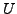per unit of mass needed to complete the phase transition satisfies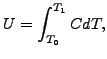(310)

where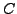is the specific heat and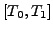is the temperature interval within which the phase transition takes place.Next: Acoustics Up: Types of analysis Previous: Direct integration dynamic analysis   Contents
guido dhondt 2018-12-15